Processing ......FreeComputerBooks.com Links to Free Computer, Mathematics, Technical Books all over the World

Quantum Computing
Related Book Categories:
•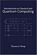Introduction to Classical and Quantum Computing (Tom Wong)

This book is for students who want to learn quantum computing beyond a conceptual level, but who lack advanced training in mathematics. The only prerequisite is trigonometry, and mathematics beyond that will be covered.

•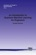An Introduction to Quantum Machine Learning for Engineers

This book provides a self-contained introduction to Quantum Machine Learning for an audience of engineers with a background in probability and linear algebra, describes the necessary background, concepts, and tools, covers parametrized quantum circuits, etc.

•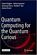Quantum Computing for the Quantum Curious

This open access book makes quantum computing more accessible than ever before. It bridges the gap between popular science articles and advanced textbooks by making key ideas accessible with just high school physics as a prerequisite.

•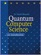Quantum Computer Science: An Introduction (David Mermin)

Quantum Physics has some spectacular applications in computer science. This book is a concise introduction to Quantum Computation, developing the basic elements of this new branch of computational theory without assuming any background in physics.

•Learn Quantum Computation using Qiskit

This is a free digital textbook that will teach you the concepts of quantum computing while you learn to use the Qiskit SDK. It is built on a jupyter notebook framework that allows for easy reading, but it also allows readers to edit and run the code right in the textbook.

•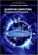Quantum Computing: Progress and Prospects

Provides an introduction to the Quantum Computing, including the unique characteristics and constraints of the technology, and assesses the feasibility and implications of creating a functional quantum computer capable of addressing real-world problems.

•Quantum Computing Since Democritus (Scott Aaronson)

This book takes readers on a tour through some of the deepest ideas of maths, computer science and physics. Full of insights, arguments and philosophical perspectives, the book covers an amazing array of topics.

•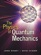The Physics of Quantum Mechanics (James Binney, et al.)

This book aims to give students a good understanding of how quantum mechanics describes the material world. It shows that the theory follows naturally from the use of probability amplitudes to derive probabilities.

•Mathematical Foundations of Quantum Theory

This is a collection of lecture notes, all shared a common interest in answering quantum issues. The goal is to give a mathematically clear and self-containing explanation of the main concepts of the modern language of quantum theory.

•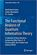The Functional Analysis of Quantum Information Theory

This book provides readers with a concise introduction to current studies on operator-algebras and their generalizations, operator spaces and operator systems, with a special focus on their application in Quantum Information Science.

Book Categories
 :All CategoriesRecent BooksMiscellaneous BooksComputer LanguagesComputer ScienceData Science/DatabasesElectrical EngineeringJava and Java EE (J2EE)Linux and UnixMathematicsMicrosoft and .NETMobile ComputingNetworking and CommunicationsSoftware EngineeringSpecial TopicsWeb Programming
Other Categories